Question

# Assume that a sample is used to estimate a population proportion p. Find the 95% confidence...

Assume that a sample is used to estimate a population proportion p. Find the 95% confidence interval for a sample of size 266 with 82 successes. Enter your answer as a tri-linear inequality using decimals (not percents) accurate to three decimal places.

_______ < p < ______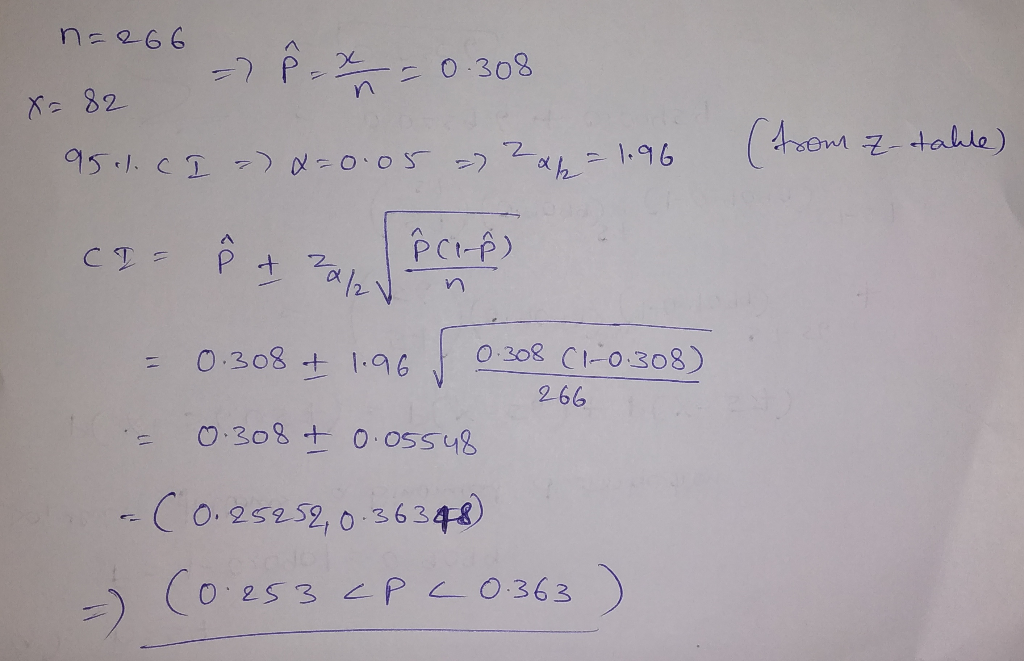#### Earn Coins

Coins can be redeemed for fabulous gifts.

Similar Homework Help Questions
• ### Assume that a sample is used to estimate a population proportion p. Find the 95% confidence...

Assume that a sample is used to estimate a population proportion p. Find the 95% confidence interval for a sample of size 186 with 56% successes. Enter your answer as an open-interval (i.e., parentheses) using decimals (not percents) accurate to three decimal places. Confidence interval = Express the same answer as a tri-linear inequality using decimals (not percents) accurate to three decimal places. < p < Express the same answer using the point estimate and margin of error. Give your...

• ### Assume that a sample is used to estimate a population proportion p. Find the 80% confidence...

Assume that a sample is used to estimate a population proportion p. Find the 80% confidence interval for a sample of size 227 with 112 successes. Enter your answer as a tri-linear inequality using decimals (not percents) accurate to three decimal places. ____< p < ____

• ### Assume that a sample is used to estimate a population proportion p. Find the 90% confidence...

Assume that a sample is used to estimate a population proportion p. Find the 90% confidence interval for a sample of size 248 with 120 successes. Enter your answer as a tri-linear inequality using decimals (not percents) accurate to three decimal places. _________< p <__________

• ### Assume that a sample is used to estimate a population proportion p. Find the 99.5% confidence...

Assume that a sample is used to estimate a population proportion p. Find the 99.5% confidence interval for a sample of size 82 with 73 successes. Enter your answer as a tri-linear inequality using decimals (not percents) accurate to three decimal places. < p < Answer should be obtained without any preliminary rounding. However, the critical value may be rounded to 3 decimal places.

• ### Assume that a sample is used to estimate a population proportion p. Find the 90% confidence...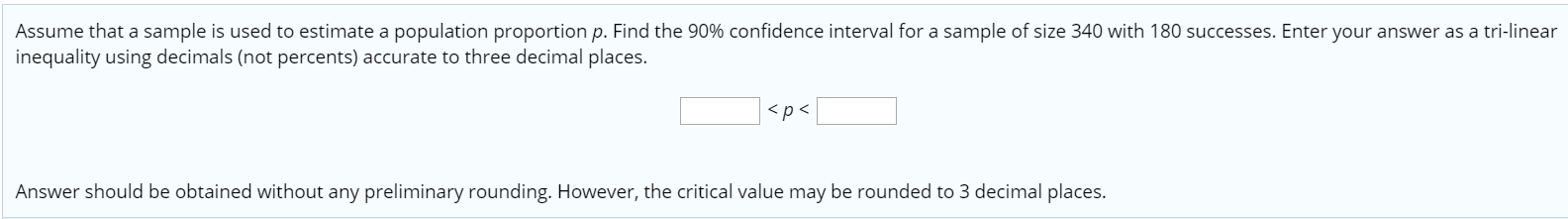Assume that a sample is used to estimate a population proportion p. Find the 90% confidence interval for a sample of size 340 with 180 successes. Enter your answer as a tri-linear inequality using decimals (not percents) accurate to three decimal places. <p> Answer should be obtained without any preliminary rounding. However, the critical value may be rounded to 3 decimal places.

• ### Assume that a sample is used to estimate a population proportion p. Find the 99% confidence...

Assume that a sample is used to estimate a population proportion p. Find the 99% confidence interval for a sample of size 308 with 123 successes. Enter your answer as a tri-linear inequality using decimals (not percents) accurate to three decimal places. < p < Answer should be obtained without any preliminary rounding. However, the critical value may be rounded to 3 decimal places.

• ### Assume that a sample is used to estimate a population proportion p. Find the 80% confidence...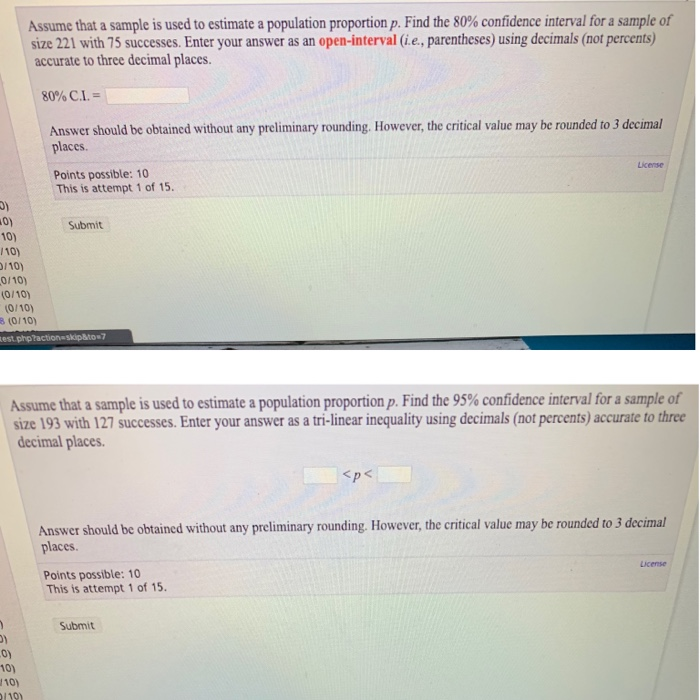Assume that a sample is used to estimate a population proportion p. Find the 80% confidence interval for a sample of size 221 with 75 successes. Enter your answer as an open-interval (i.e., parentheses) using decimals (not percents) accurate to three decimal places. 80% C.L. - Answer should be obtained without any preliminary rounding. However, the critical value may be rounded to 3 decimal places License Points possible: 10 This is attempt 1 of 15. Submit 10) 110) 1/10) 0/10)...

• ### Assume that a sample is used to estimate a population proportion p. Find the 95% confidence...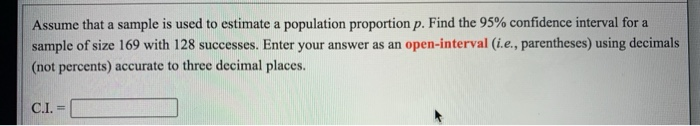Assume that a sample is used to estimate a population proportion p. Find the 95% confidence interval for a sample of size 169 with 128 successes. Enter your answer as an open-interval (i.e., parentheses) using decimals (not percents) accurate to three decimal places. C.I.

• ### Assume that a sample is used to estimate a population proportion p. Find the 99.9% confidence...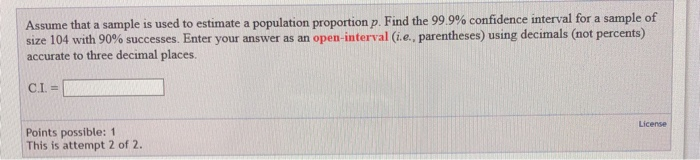Assume that a sample is used to estimate a population proportion p. Find the 99.9% confidence interval for a sample of size 104 with 90% successes. Enter your answer as an open-interval (i.e., parentheses) using decimals (not percents) accurate to three decimal places. C.I.= License Points possible: 1 This is attempt 2 of 2. Assume that a sample is used to estimate a population proportion p. Find the 99.9% confidence interval for a sample of size 104 with 90% successes....

• ### Assume that a sample is used to estimate a population proportion p. Find the 95% confidence interval for a sample of siz...

Assume that a sample is used to estimate a population proportion p. Find the 95% confidence interval for a sample of size 155 with 20 successes. Enter your answer as an open-interval (i.e., parentheses) using decimals (not percents) accurate to three decimal places. 95% C.I. = Answer should be obtained without any preliminary rounding. However, the critical value may be rounded to 3 decimal places.